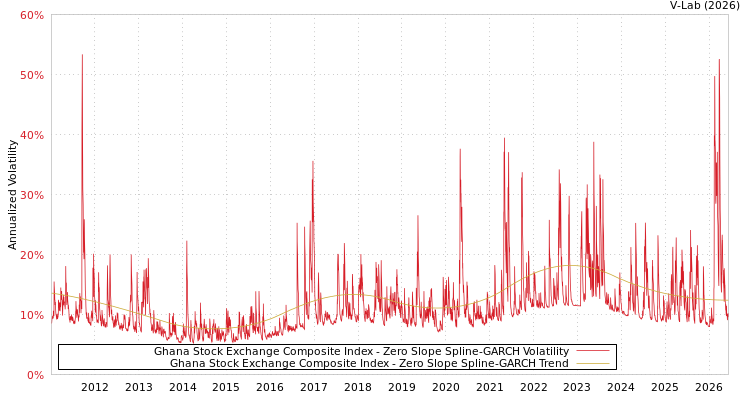##### Ghana Stock Exchange Composite Index Zero Slope Spline-GARCH Volatility Analysis
###### Volatility Prediction for Monday, September 25th, 2023:14.05% (-0.19%)

Analysis last updated: Saturday, September 23, 2023 at 04:35 AM UTC

###### Date Range:

from

to

6M ·

1Y ·

2Y ·

5Y ·

10Y ·

Allparamt-stat
$\omega$1.05684.28
$\alpha$0.12865.03
$\beta$0.750013.95
${\gamma }_{1}$-0.0816-0.85
${\gamma }_{2}$0.28641.91
${\gamma }_{3}$-0.3788-2.78
${\gamma }_{4}$0.33302.39
${\gamma }_{5}$-0.2572-2.66
Estimation Period:
Jan 4, 2011 to Sep 22, 2023